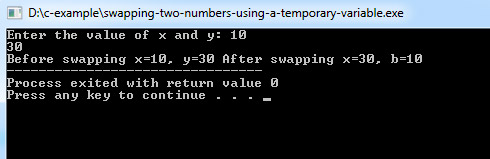# C Program to Swapping Two Numbers Using a Temporary Variable

This C program is used to swapping two numbers, using a temporary variable.

Example:
``````#include<stdio.h>

int main() {
int x, y, temp;
printf("Enter the value of x and y: ");
scanf("%d %d", &x, &y);
printf("Before swapping x=%d, y=%d ", x, y);

/*Swapping logic */   temp = x;
x = y;
y = temp;
printf("After swapping x=%d, b=%d", x, y);
return 0;
}``````
Program Output:Explanation:

This program is used to swap values of two variables using the third variable, which is the temporary variable. So first of all, you have to include the stdio header file using the "include" preceding by # which tells that the header file needs to be process before compilation, hence named preprocessor directive.

Then you have to define the main() function and it has been declared nothing so by default it  returns integer. Now, you have to first take three integer type variable name as 'x', 'y' and 'temp'. Then you have to use the printf() function to display this message - "Enter the value of x and y: ". The scanf will take the value of 'x' and 'y' from the user using the scanf(). Again, another printf() is used to display the value of 'x' and 'y' before swapping.

Then for swapping, the value of 'x' get stored in 'temp', then the value of 'y' is stored in 'x' and lastly the value of 'temp' is stored to 'x'. This is how the value of 'x' get changed to the value of 'y'.a

Another printf() function is used to display the new swapped value of 'x' and 'y'. In the end, the return 0; statement is used to return an integer type value back to main().